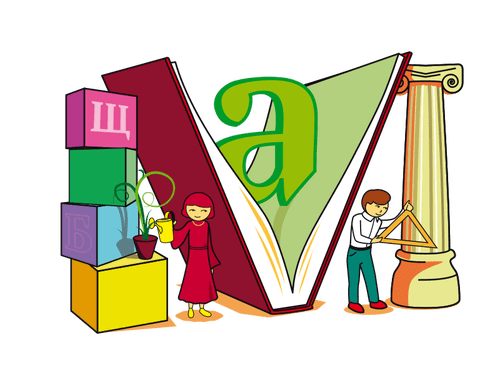### Math exercises and problems

This section represents a collection of exercises with clues and examples which are meant to help you better understand basic concepts. As opposed, when solving exercises from your homework, you won’t be given any clues.

You can practice math for free from Kidergarten to Eight grade.

### Math Homework

The homework section is useful for parents or teachers to put their children to work. They know exactly what exercises the children encounter. You can select which exercise and how many times to solve it.

### Online Math Tests

The test section is useful for evaluating the math skills. At the end of each test, you will receive a note depending on how many exercises you have solved correctly.Site-ul nostru poate rămâne gratuit numai prin afișarea de reclame.

Vă rugăm să ne susțineți prin dezactivarea blocării de anunțuri.